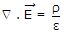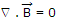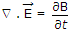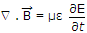# Electronics and Communication Engineering - Exam Questions Papers

1.
If RT, Rh represent condition for reciprocity in case of transmission parameter and hybrid parameter respectively and similarly ST, Sh represent condition for symmetry in case of transmission parameter and hybrid parameter respectively then match the following :
(1) RT (a) A = D
(2) Rh (b) h12 = h21
(3) ST (c) AD - BC = 1
(4) Sh (d) h12 = - h21
(e) B = c
(f) Δh = 1
1 - c 2 - d 3 - a 4 - f
1 - c 2 - b 3 - e 4 - d
1 - e 2 - d 3 - a 4 - b
1 - e 2 - d 3 - a 4 - f
Explanation:
No answer description is available. Let's discuss.

2.
The Nyquist plot of a stable, transfer function G(s) is shown in the figure. We are interested in the stability of the closed loop system in the feedback configuration shown.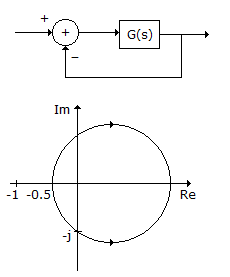The gain and phase margins of G(s) for closed loop stability are
6 dB and 180°
3 dB and 180°
6 dB and 90°
3 dB and 90°
Explanation:
No answer description is available. Let's discuss.

3.
The eigen values of the following matrix are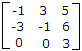3, 3 + 5j, 5 -j
- 6 + 5j, 3 + j, 3 - j
3 + j, 3 - j, 5 + j
3, - 1 + 3j, - 1 - 3j
Explanation:
No answer description is available. Let's discuss.

4.
Faraday's induction law states that a changing magnetic field is accompanied by an electric field. Which of the following equation represents it?